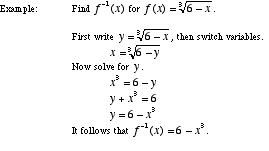index: click on a letter A B C D E F G H I J K L M N O P Q R S T U V W X Y Z A to Z index index: subject areas numbers & symbols sets, logic, proofs geometry algebra trigonometry advanced algebra & pre-calculus calculus advanced topics probability & statistics real world applications multimedia entrieswww.mathwords.com about mathwords website feedback

 Inverse Function The function obtained by switching the x- and y-variables in a function. The inverse of function f is written f -1. Note: The new relation obtained by reversing the x- and y-values of a function is not necessarily a function itself. The new relation is only a function if the original function is one-to-one.